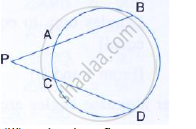Share

# (Ii) in the Given Figure, 5 × Pa = 3 × Ab = 30 Cm and Pc = 4cm. Find Cd. - Mathematics

Course

#### Question

(ii) In the given figure, 5 × PA = 3 × AB = 30 cm and PC = 4cm. find CD.#### Solution

Since two chords AB and CD intersect each other at P.
∴ AP ×  PB =  CP × PD
But 5 × PA = 3 × AB = 30 cm
∴ 5× PA = 30cm ⇒ PA =  6cm
And 3× AB =30cm ⇒ AB = 10cm

⇒ BP = PA + AB  = 6 + 10 = 16 cm

Now,
AP × PB = CP  PD
⇒ 6 × 16 = 4 ×PD
⇒ PD = (6×16)/4 =  24 cm
CD = PD – PC = 24 – 4 = 20 cm

Is there an error in this question or solution?